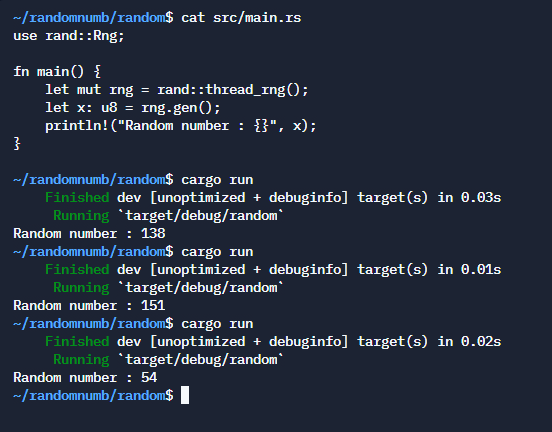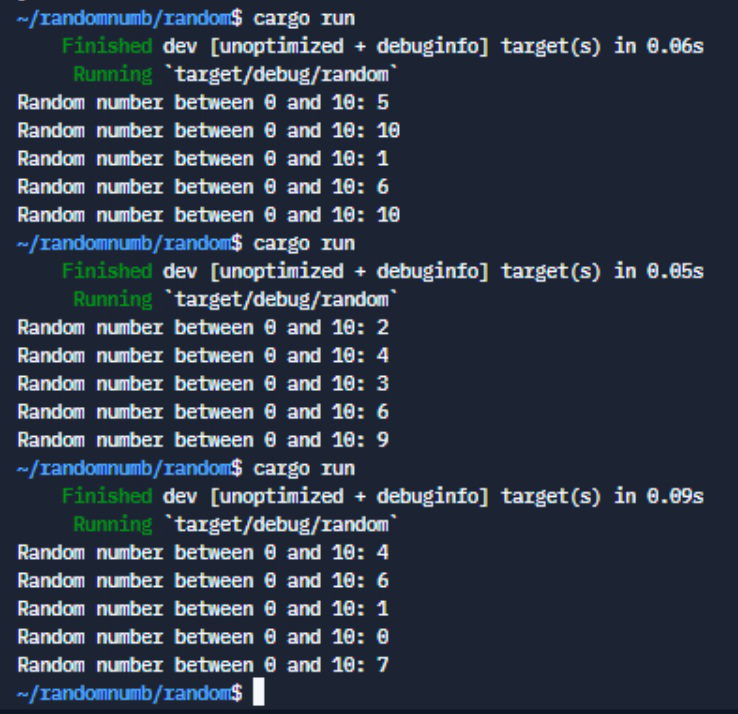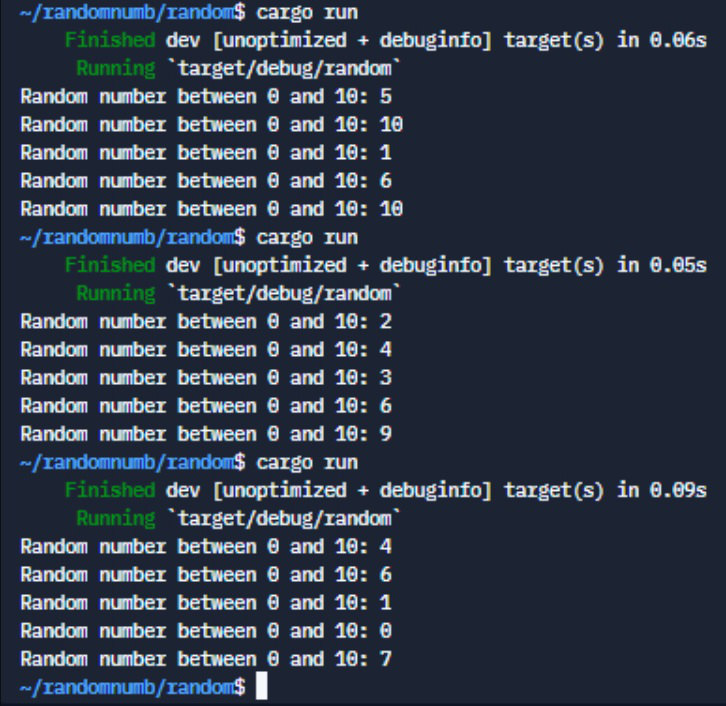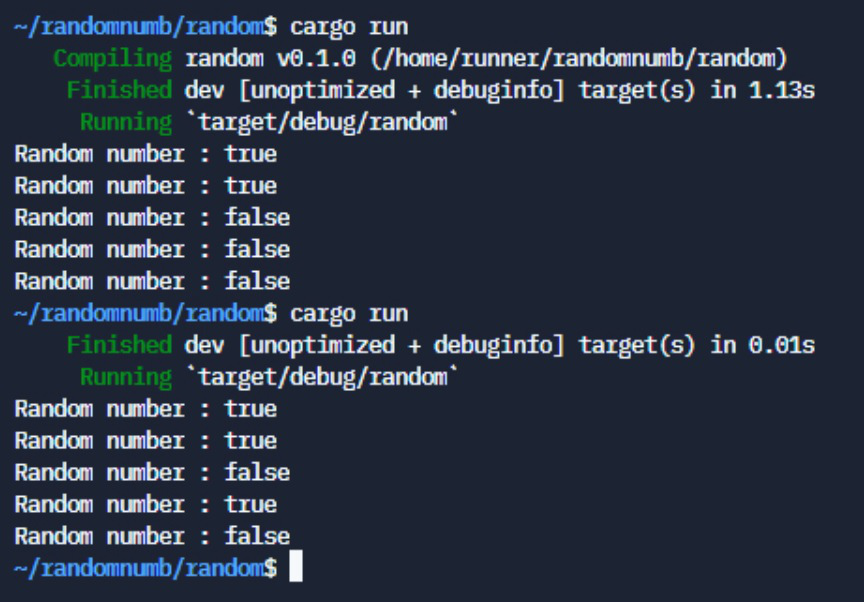# How to Generate Random Numbers in Rust Language?

• Last Updated : 28 Apr, 2022

Random number generation is an important feature in programming to design complex and automated programs. We can generate random numbers in rust with the help of the rand crate.

### Generate a Random Number:

We will use the rand crate and the Rng trait in Rust that gives us access to certain functions that help us in generating random numbers.  Inside the main function, we can then create a mutable rng variable which we will assign as a random number generator that is needed by the system (random value from which the thread will assign numbers). Using the rng thread, we can generate a random number appropriate to the limit of the variable assigned.

Using the gen function which returns the number within the bounds of the variable type in this vase u8, the number returned is given out by a standard distribution.We can even generate random numbers beyond the 8-bit like, u16, u32, u64, u128, or even signed integers with i8, i16, i32, i64, and i128.

### Generate Random Numbers within a Range:

We can also generate random numbers with a specified range. We use the gen_range function to get a random number by uniform distribution between the two limits passed as its parameter. To demonstrate this, we run a for loop and print the number generated from the gen_range function in each iteration.

`rng.gen_range(0..10)`

The upper bound is non-inclusive so the number 10 won’t be generated from the function. However, we can make it inclusive by adding the = operator before the upper bound.

`rng.gen_range(0..=10)`

Example 1:

## Rust

 `// RUST code: Generate Random Numbers within a Range``use` `rand``::Rng;`` ` `fn` `main() {``    ``let` `mut` `rng = ``rand``::thread_rng();``     ` `    ``for` `_x ``in` `0..5{``    ``let` `num: u8 = rng.gen_range(0..10);``    ``println!(``"Random number between 0 and 9: {}"``, num);``    ``}``}`

Output:Example 2:

## Rust

 `// RUST Code: Using the inclusive range ``// for generating random numbers``use` `rand``::Rng;`` ` `fn` `main() {``    ``let` `mut` `rng = ``rand``::thread_rng();``     ` `    ``for` `_x ``in` `0..5{``    ``let` `num: u8 = rng.gen_range(0..=10);``    ``println!(``"Random number : {}"``, num);``    ``}``}`

Output:In this example, we were able to generate random numbers within a given range both inclusive and non-inclusive upper bound.

### Generate Random Boolean:

We can even generate random boolean values with a species distribution of the probabilities of true and false. Using the gen_bool function and passing the distribution as a fraction to it we get a boolean value from the function.

Example 3:

## Rust

 `// RUST Code: Generating random boolean value``use` `rand``::Rng;`` ` `fn` `main() {``    ``let` `mut` `rng = ``rand``::thread_rng();``     ` `    ``for` `_x ``in` `0..5{``    ``let` `toss: ``bool` `= rng.gen_bool(0.5);``    ``println!(``"Random number : {}"``, toss);``    ``}``}`

Output:As we have given a parameter of 0.5 it will generate the boolean values evenly, if we give the distribution as 1, it will only print true as the probability of false or other event becomes 0.

Thus,  we were able to generate random numbers in Rust using the rand create with gen, gen_range, and gen_bool methods to generate numbers in different distributions.

My Personal Notes arrow_drop_up